Courses

# Test: Square Root And Cube Root- 2

## 10 Questions MCQ Test General Aptitude for GATE 2020 | Test: Square Root And Cube Root- 2

Description
This mock test of Test: Square Root And Cube Root- 2 for UPSC helps you for every UPSC entrance exam. This contains 10 Multiple Choice Questions for UPSC Test: Square Root And Cube Root- 2 (mcq) to study with solutions a complete question bank. The solved questions answers in this Test: Square Root And Cube Root- 2 quiz give you a good mix of easy questions and tough questions. UPSC students definitely take this Test: Square Root And Cube Root- 2 exercise for a better result in the exam. You can find other Test: Square Root And Cube Root- 2 extra questions, long questions & short questions for UPSC on EduRev as well by searching above.
QUESTION: 1

### How many two-digit numbers satisfy this property : The last digit (units digit) of the square of the two-digit number is 8 ?

Solution:

A number ending in 8 can never be a perfect square.
12 = 1
22 = 4
32 = 9
42 = 16
52 = 25
62 = 36
72 = 49
82 = 64
92 = 81

QUESTION: 2

### if a = 0.2917, then the value of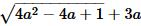is :

Solution: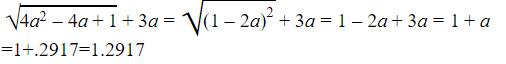QUESTION: 3

### A group of students decided to collect as many paise from each member of group as is the number of members. If the total collection amounts to Rs. 59.29, the number of the member is the group is:

Solution:

Money collected = (59.29 x 100) paise = 5929 paise.
∴ Number of members = √5929 = 77.

QUESTION: 4

if √7 = 2.645, then find the value of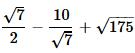Solution: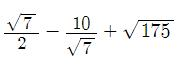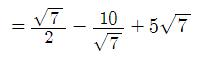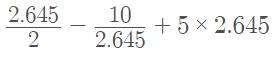= 1.3225 - 3.78071 + 13.225
= 10.76679 ≈ 10.77

QUESTION: 5

What is smallest number with which 5400 may be multiplied so that the product is perfect cube?

Solution:

Find prime factors of 5400

5400=2×3×3×3×2×2×5×5

If we group them in the group of 3

5400=(2×2×2)×(3×3×3)×5×5

Here to make group of 3 for 5

We have to multiply 5400 by 5.

QUESTION: 6

The square root of (14 + 2√13)(14 - 2√13) is

Solution:

As we know that  (a²-b²) = (a+b) (a-b)
Therefore 14² - 2²x13 = 144.
So √144 = 12.

QUESTION: 7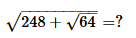Solution:

= [√248+√64]
= √248+8
= √256
= 16

QUESTION: 8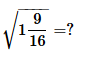Solution:

√1 9/16
= √25/16
= 5/4
= 1 1/4.

QUESTION: 9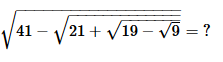Solution:

√41-√21+√19-√9
= √41-√21+√19-3
=√41-√21+√16
=√41-√21+4
=√41-√25
=√41-5
=√36
=6

QUESTION: 10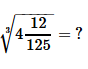Solution:

Cube root of (512)/125
= 8/5
Convert it into mixed fraction, we get
= 1 3/5